﻿ Validation of Statistically Derived Estimated Models for Experimental Investigation of Pico Hydro Pelton Turbine CupPublications are Open
Access in this journal
Article Versions
Export Article
• Normal Style
• MLA Style
• APA Style
• Chicago Style
Research Article
Open Access Peer-reviewed

### Validation of Statistically Derived Estimated Models for Experimental Investigation of Pico Hydro Pelton Turbine Cup

Fapetu Oluseyi Feyisetan, Akinola Adebisi Olayinka, Akintunde Mutalubi Aremu, Raimi Oluwole Abiodun , Adesina Fisayo
Applied Mathematics and Physics. 2017, 5(2), 53-60. DOI: 10.12691/amp-5-2-4
Published online: June 01, 2017

### Abstract

Theoretical force plays an important role in the design of hydro power system when comparing it with the experimental force data which is a function of rate of momentum in jet while no significant reference is given to the estimated force which is statistically derived and also a factor of the experimental force data. The aim of the paper is to statistically derive an estimated model for the force in the Pico hydro turbine cup which will be an alternative for theoretical force in the experimental investigation. Data in the experiment were taken and recorded for six (6) different observations of added weight of mass of 2.5kg, 4.0kg, 6.0kg, 8.0kg and 10.0kg to quantity of mass of 7.5kg, 12.0kg, 18.0kg, 24.0kg and 30.0kg respectively. Regression analysis was the statistical tool employed with the aid of Minitab 16 software to get the estimated force for the variation of added weight of mass. The findings revealed that the estimated model for force on cup displays very significant output when compared with the theoretical force. It is concluded that the estimated force model can be an alternative for theoretical force in an experimental investigation of Pico hydro pelton turbine cup as long as it is specified within the limit of quantity of mass used.

### 1. Introduction

The benefit of hydro power systems in Nigerian has been given a lot of consideration in terms of its size, cost, simplicity in the design approach, planning and installation. The maximum electricity output of five kilowatts (5KW) of hydro power is known as Pico hydro. Recent innovations in Pico-hydro technology have made it an economic source of power even in some of the world’s poorest and most inaccessible places 1. In designing, developing and experimenting on hydro power systems, there is proper need to validate the experimental investigation through formulated models. Model validation has been so important for every experimental study. To ascertain if an experimental inference conforms to a theoretical inference brings a significant deduction to any research findings.

Most research study in areas of Pico hydro system gives little or no attention to validate the experimental design. Edeoja et al., 2 conceptually designed a simple decentralized self-powering pico hydro power system which uses the basic principle of a pumped storage hydro system without proper validation of the design through experimental investigation. Zainuddin et al., 1 described only the design and development of pico-hydro generation system using consuming water distributed to houses without validating the process.

Model validation is obviously carried out in almost any research study that involves experimental investigation. Thakur and Chauhan 3 validated an experimental result that shows that the coefficient of friction and specific wear rate decreased with the increase in applied normal load and sliding speed using the morphologies of the worn surfaces by means of scanning electron microscope (SEM). The validation showed a good agreement with the experimental result. In the study of Nomex honeycombs under combined shear-compression with regard to out-of-plane direction, Zhou et al., 4 presented an experimental investigation on the yield behaviour. The experimental results of the study validated that the comparative analytical results indicated in the experimental yields surfaces were approximately consistent with the theoretical yield surfaces in the normal-shear stress space.

Li et al., 5 presented a study on the modelling and parametric analysis of recently proposed nonlinear compressive-mode energy harvester under harmonic excitation. Both theoretical and experimental investigations were performed in the study over a range of excitation frequencies. Based on the study findings, it is observed that the analytical model is well validated against the experimental data through a good agreement which was achieved.

Driss et al., 6 developed a meshing effect on the numerical results of a NACA2415 airfoil wind turbine placed in an open wind tunnel using a commercial CFD code based on the resolution of the Navier-Stokes equations n conjunction with the standard k-ε turbulence model. The developed numerical results were compared with the experimental results to choose the adequate meshing and which in turn showed a good agreement.

Casano et al., 7 studied the fluid dynamics performance of electronic equipment designed to cool a heat sink in the form of a finned duct. The experimental investigation revealed that the experimental working conditions of the active fans were consistent with the manufacturers’ characteristic curves. This implies that the experimental working points agreed very well with the theoretical curve of the pressure drop in the study.

Cepon et al., 8 performed the validation of a developed belt-drive model using a two-pulley belt-drive. The numerically obtained results when compared with the experimental results under various operational conditions showed a good agreement. It is in view of this that the paper seeks to investigate experimentally the validity of the statistically derived estimated models for the Pico hydro pelton turbine cup.

### 2. Materials and Methods

The apparatus for the experiment are Timer, Volumetric Hydraulic Bench H1D (Tec-Quipment), Impact of jet apparatus (H8) (Tec-Quipment), Jockey weight and gravimetric weights. The experiment is set up such that the balance beam is horizontal when the upward force of the water impinging on the target is balanced by the downward force of the jockey weight. This means that the balance beam and the target are considered to be weightless. To achieve this weightless estate, the beam is levelled by changing the tension of the adjusting spring shown in the Figure 1 when no water is flowing and for the jockey mass at x1 = 0. This must be done for each target used as they are not expected to have the same weight. In taking data, it would be convenient to have round values of x2. Thus, the water flow was turned on full force, the jockey weight positioned to the nearest round value, and the water flow backed off just enough to level the balance bar. (It is easier to adjust the water flow for a given position of the jockey weight as to trying to position of the jockey weight for a specified flow of water.). At the left of the schematic is shown a weight hanger. It is quite heavy in its own right-even before additional weight is added. The discharge hopper (weighing tank) is emptied until the weight hanger drops under its own weight. The drain plug at the bottom of the tank is reinserted and the tank begins to fill. At the point in filling the tank when the tank balance bar and weight hanger rise up and hit a stop, a stop watch is started. Directly after starting the watch, 2.5kg, 4.0kg, 6.0kg and 10.0kg of additional weight is added to the weight hanger of 7.5kg, 12.0kg, 18.0kg, 24.0kg and 30.0kg respectively. As the discharge tank continues to fill, eventually enough weight of water is achieved to outweigh the 2.5kg, 4.0kg, 6.0kg and 10.0kg of additional weight that was respectively added. This causes the tank balance beam to rise up against the stop again at which time the stop watch is halted. The ratio of moment arms on either side of the fulcrum of the tank balance beam is 3:1 such that 2.5kg, 4.0kg, 6.0kg and 10.0kg of additional weight is respectively balanced by 7.5kg, 12.0kg, 18.0kg, 24.0kg and 30.0kg of water. The flow rate is then 7.5kg, 12.0kg, 18.0kg, 24.0kg and 30.0kg of water during the time that the stop watch was running. Decreasing values of x2 are then laid in and flow reduced accordingly.

The technical details used for the computation are: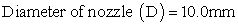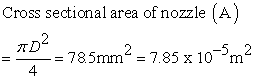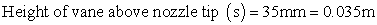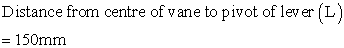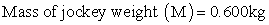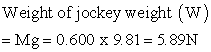When there is a change in distance,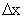of the movement of the jockey weight from its zero position, the force F on the vane which is required to restore balance is given by: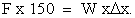Substituting 5.98N for W gives: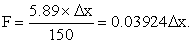(1)

The mass flow rate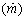of the jet is computed by timing the collection of a known mass of water. The velocity U1 of the jet as it leaves the nozzle was obtained from the volumetric flow rate Q and the cross sectional area A of the nozzle. The velocity U0 with which the jet strikes the vane was slightly less than U1 because of the deceleration due to gravity. This reduction in velocity can be determined using Bernoulli's equation,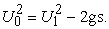(2)

In equation (13), g = 9.81 m/s2 is the local value of the gravitational acceleration while s is the distance between the nozzle outlet and the vane. The nozzle velocity was determined from the mass flow rate as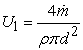(3)

Where ρ is the water density and d is the nozzle diameter.

Readings were taken for quantity collected, measured time, jockey displacement, mass flow rate, initial velocity U1 and final velocity U0.

• Figure 1. Schematic Diagram of Volumetric hydraulic bench and experiments processes 
2.1. Theoretical Model

Considering the equation of momentum and how it applies to the case shown schematically in Figure 2, which shows a jet of fluid impinging on a symmetrical vane.

• Figure 2. Sketch of jet impinging on a vane

Let:

Mass flow rate in the jet =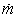Control volume = V

Control surface which encloses the vane = S

The velocity with which the jet enters the control volume in the x-direction = U1.

The jet is deflected by its impingement on the vane, so that it leaves the control volume with velocity = U0 which is inclined at an angle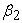to the x-direction.

Now the pressure over the whole surface of the jet, apart from that part where it flows over the surface of the vane, is atmospheric. Therefore, neglecting the effect of gravity, the changed direction of the jet is due solely the force generated by pressure and shear stress at the vane's surface. If this force on the jet in the direction of x be denoted by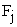, then the momentum equation in the x-direction is: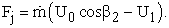(4)

Equation (4) is the force on the vane and is equal and opposite to equation (5):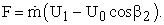(5)

For the case of a flat plate,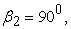so that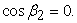It follows that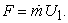(6)

Equation (6) is the force on the flat plate, irrespective of the value of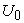.

For the case of a hemispherical cup, we assume that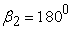, so that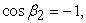and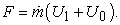(7)

If we neglect the effect of change of elevation on jet speed, and the loss of speed due to friction over the surface of the vane, then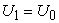, so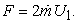(8)

Equation (8) is the maximum possible value of force on the hemispherical cup. This is just twice the force on the flat plate.

Returning now to Figure 2, the rate of at which momentum is entering the control is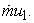we can also suggest this as a rate of flow of momentum in the jet, and denote it by the symbol J, where: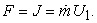(9)

For the flat plate,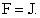And for the hemispherical cup, the maximum possible value of force is: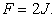(10)
2.2. Estimated Model

The estimated model is a linear regression model formulated from the experimental set up that forms the research paper. The estimated model is formulated for jet impact on hemispherical vane with added mass of 2.5kg, 4.0kg, 6.0kg, 8.0kg and 10.0kg for quantity of mass of 7.5kg, 12.0kg, 18.0kg, 24.0kg and 30.0kg respectively.

Model 1 for added mass weight of 2.5kg: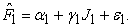(11)

Model 2 for added mass weight of 4.0kg: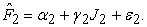(12)

Model 3 for added mass weight of 6.0kg: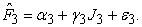(13)

Model 4 for added mass weight of 8.0kg: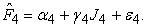(14)

Model 5 for added mass weight of 10.0kg: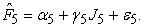(15)

Where: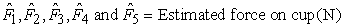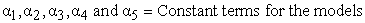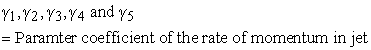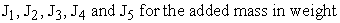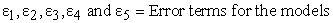### 3. Results and Discussion

Data were taken for a total of six (approximately evenly spaced) positions of the jockey weight as shown on Table 1, Table 2, Table 3, Table 4 and Table 5. The aim of the study is to verify if the theoretical model is in agreement with the statistically derived estimated model of the investigated Pico hydro pelton turbine cup.

• Figure 3. Relationship between Estimated and Theoretical value of Force on Cup for Added Mass Weight 2.5kg
• Figure 4. Relationship between Estimated and Theoretical value of Force on Cup for Added Mass Weight 4.0kg
• Figure 5. Relationship between Estimated and Theoretical value of Force on Cup for Added Mass Weight 6.0kg
• Figure 6. Relationship between Estimated and Theoretical value of Force on Cup for Added Mass Weight 8.0kg
• Figure 7. Relationship between Estimated and Theoretical value of Force on Cup for Added Mass Weight 10.0kg

The estimated models were analyzed using regression techniques for Table 1, Table 2, Table 3, Table 4 and Table 5, where force experimental (F) serves as the dependent variable and the rate of momentum on jet (J) serves as the independent variable. Table 6 shows the summary of the regression analysis for the estimated models for added mass weight of 2.5kg, 4.0kg, 6.0kg, 8.0kg and 10.0kg to quantity of mass of 7.5kg, 12.0kg, 18.0kg, 24.0kg and 30.0kg respectively (detailed regression output is shown in Appendix A). The coefficient of determination (R2) for the models was significantly high 10 and which stood at 94.1%, 97.0%, 98.5%, 97.9% and 98.0%. The value implies that the estimated forces on cup are better explained by the rate of momentum in jet while the 5.9%, 3.0%, 1.8%, 2.1% and 2.0% are the error terms that are unforeseen in the experiment. The adjusted R2 of 92.7%, 96.2%, 98.2%, 97.3% and 97.5% depicted the true behaviour of the R2. However, the formulated models show a good fit 11, 12.

Also, the probability values of 0.001, 0.000, 0.000, 0.000, 0.000 for the added mass weight of 2.5kg, 4.0kg, 6.0kg, 8.0kg and 10kg against the F-statistic values of 64.31, 128.84, 266.30, 184.63 and 196.51 was lesser than the test of significance at 0.05; this was also in agreement with the R2 13 that the formulated models are good predictor of the forces on cup within the specified boundary of the added weight of mass used in the experimental investigation. This results shows similar approach to some research study 14, 15 and the tests constitute a good sample of the range of applicability of small scale turbines as valid solutions for micro-hydro 14.

Graphical analysis was also introduced to ascertain the level of agreement between the theoretical model and estimated model for the forces on cup using Table 7. Figure 3, Figure 4, Figure 5, Figure 6 and Figure 7 shows the relationship between the estimated and theoretical value of force on cup for added mass weight of 2.5kg, 4.0kg, 6.0kg, 8.0kg and 10.0kg. For added weight of mass of 2.5kg and 4.0kg to 7.5kg and 12.0kg quantity of mass, both the estimated (FEM) and theoretical (FTM) line graphs maintained the same level for the number of observations considered, Figure 3 and Figure 4. Also, for added mass weight of 6.0kg, 8.0kg and 10kg to 18.0kg, 24.0kg and 30.0kg quantity of mass, the graphs showed that there were perfect uniform stability for added weight of 6.0kg, 8.0kg and 10.0kg between 4th and 6th observations, despite the dramatic movement between 1st and 3rd observations, Figure 4, Figure 5, Figure 6 and Figure 7. However, the dramatic movement had no negative effect to the estimated force model because the overriding high effect of the R2 had corrected that through a very low value of error terms (Table 6) been displayed by the number of observations in the experiment been considered.

Figure 3, Figure 4, Figure 5, Figure 6 and Figure 7 depicted the true nature of the experimental data of the force on cup through the estimated and theoretical values on the graph. This implies that the value of the force theoretical can also be derived statistically from an estimated model based on the experimental design and structure of the investigation.

### 4. Conclusion

The respective models as for the added mass weight of 2.5kg, 4.0kg, 6.0kg, 8.0kg and 10kg to quantity of mass of 7.5kg, 12.0kg, 18.0kg, 24.0kg and 30.0kg respectively, described in this paper study can predict accurately the forces on cup based on the rate of momentum in jet within the limit of the experimental data. The models displayed a very high coefficient of determination (R2) that tells us how significant the models are in term of prediction of the forces for Pico Hydro turbine cup. The models would help researchers to look into some design considerations when fabricating turbine cup with locally raw material against the exact proportion of added mass weight ratio of the forces on cup so as to enhance the efficiency. It can generally be concluded that these models show good agreement with the experimental results.

### References

  H. Zainuddin, M.S. Yahaya, J.M. Lazi, M.F.M. Basar, and Z. Ibrahim, Design and development of Pico-hydro generation system for energy storage using consuming water distributed to houses. International Journal of Electrical, Computer, Energetic, Electronic and Communication Engineering, 3(11), 2009, 1922-1927. In article View Article  A.O. Edeoja, S.J. Ibrahim, and E.I. Kucha, Conceptual design of a simplified decentralized Pico hydropower with provision for recycling water. Journal of Multidisciplinary Engineering Science and Technology, 2(2), 2015, 22-34. In article View Article  S. Thakur, and S.R. Chauhan, Experimental investigation of cenosphere particulate filled E-glass reinforced vinylester composites under dry and water lubricated sliding conditions. Indian Journal of Engineering & Materials Sciences, 22, 2015, 669-678. In article View Article  Z. Zhou, Z. Wang, L. Zhao and X. Shu, Experimental investigation on the yield behaviour of Nomex honeycombs under combined shear-compression. Latin American Journal of Solids and Structures, 9, 2012, 515-530. In article View Article  H.T. Li, Z. Yang, J. Zu, and W.Y. Qin, Distributed parameter model and experimental validation of a compressive-mode energy harvester under harmonic excitations. American Institute of Physics (AIP), 6 (085310), 2016, 1-16. In article View Article  Z. Driss, T. Chelbi, W. Barhounmi, and A. Kaffel, and M.S. Abid, Numerical and experimental investigations on the meshing model choice of a NACA2415 airfoil wind turbine placed in an open wind tunnel. Journal of Physical Mathematics, 6(2), 2015, 1-5. In article View Article  G. Casano, M.W. Collins, and S. Piva, Experimental investigation of the fluid dynamics of a finned heat sink under operating conditions. Journal of Electronics Cooling and Thermal Control, 4, 2014, 86-95. In article View Article  G. Cepon, L. Mann, and M. Boltezar, Validation of a flexible multibody belt-drive model. Journal of Mechanical Engineering, 7(8), 2011, 539-546. In article View Article  M.K. Peter, Laboratory fluid mechanics manual, 2016, available at: www.utoledo.org. In article  L. Saunders, R. Russell, and D. Crabb, The coefficient of determination: what determines a useful R2 statistics? Investigative Ophthalmology & Visual Science (IOVS), 53(11), 2012, 6830-6832. In article View Article  PubMed  D. Hooper, J. Coughlan, and M. R. Mullen, Structural equation modelling: guidelines for determining model fit. Electronic Journal of Business Research Methods, 6(1), 2008, 53-60. In article View Article  J. Fan, and L. Huang, Goodness-of-fit tests for parametric regression models. Journal of the American Statistical Association, 96(454), 2001, 640-652. In article View Article  D.B.F. Filho, R. Paranhos, E.C. da Rocha, M. Batista, J.A. da Silva Jr, M.L. W.D. Santos, and J.G. Marino, When is statistical significance not significant? Brazilian Political Science Review (bpsr), 7(1), 2013, 31-55. In article View Article  G.A. Caxaria, D. D. e Sousa, and H.M. Ramos, Small scale hydropower: generator analysis and optimization for water supply systems. World Renewable Energy Congress, Hydropower Application (HP), 8-13 May, 2011, 1386-1393, Linköping, Sweden. In article View Article  J. Margeta, and Z. Glasnovic, Concept-H: sustainable energy supply. World Renewable Energy Congress, Hydropower Application (HP), 8-13 May, 2011, 1408-1415, Linköping, Sweden. In article View Article

### APPENDIX AThis work is licensed under a Creative Commons Attribution 4.0 International License. To view a copy of this license, visit http://creativecommons.org/licenses/by/4.0/